• 二阶常系数微分方程的通解 (一.) 二阶常系数微分方程的通解的组成： 其对应二阶常系数微分方程的通解 + 二阶常系数微分方程的特解 (二.) 构造二阶常系数微分方程的特解 形如：y′′+py′+qy=Pm(x)eαxy&amp;...
二阶常系数微分方程的通解
(一.) 二阶常系数微分方程的通解的组成：
      其对应二阶常系数微分方程的通解 +  二阶常系数微分方程的特解

(二.) 构造二阶常系数微分方程的特解

形如：$y''+py'+qy = P_{m(x)}e^{\alpha x}$  的二阶常系数微分方程。
$（ P_{m(x)}表示最高次数为m的多项式。）$
$构造：y*=Q_{(X)}e^{\alpha x}$
$\Rightarrow y*'=Q_{(X)}'e^{\alpha x}+\alpha Q_{(X)}e^{\alpha x}$,
$y*''= Q_{(X)}''e^{\alpha x}+2\alpha Q_{(X)}'e^{\alpha x} +{\alpha}^2Q_{(X)}e^{\alpha x}$

$将 y*, y*', y*'' ,代入y''+py'+qy = P_{m(x)}e^{\alpha x}$:
$\Rightarrow e^{\alpha x}[Q_{(X)}''+(2\alpha +p)Q_{(X)}'+({\alpha}^2+p\alpha+Q_{(x)}]= P_{m(x)}e^{\alpha x}$
即，$[Q_{(X)}''+(2\alpha +p)Q_{(X)}'+({\alpha}^2+p\alpha+Q_{(x)}]= P_{m(x)}$
$讨论：$
(1)   $\alpha 不是特征方程 r^2 + pr +q=0 的解$
$由Q_{(X)}''+(2\alpha +p)Q_{(X)}'+({\alpha}^2+p\alpha+Q_{(x)}=0 可构造$:
$Q_{(X)}=a_mx^m+a_{(m-1)}x^{(x-1)}\cdots a_1x+a_0$
（2)$\alpha 是特征方程 r^2 + pr +q=0 的单根$
$由Q_{(X)}''+(2\alpha +p)Q_{(X)}'=0 可构造$:
$Q_{(X)}'=a_mx^m+a_{(m-1)}x^{(x-1)}\cdots a_1x+a_0$
（3)$\alpha 是特征方程 r^2 + pr +q=0 的重根$
$由Q_{(X)}''=0 可构造$:
$Q_{(X)}''=a_mx^m+a_{(m-1)}x^{(x-1)}\cdots a_1x+a_0$
$最后，根据多项式相等，则其对应系数相等可求解$
解题步骤：
1.） 求解二阶常系数非齐次微分方程对应的齐次微分方程的通解
2.） 遇到形式为 $y''+py'+qy = P_{m(x)}e^{\alpha x}$  的二阶常系数微分方程， 构造$y*=Q_{(X)}e^{\alpha x}$
3.） $将 y*, y*', y*'' ,代入y''+py'+qy = P_{m(x)}e^{\alpha x}并化简$
4.） 判断 $\alpha$ 是否为特征方程的根？单根？重根？
5. ）根据 $\alpha$ 确定所构造的多项式次数并求解。

形如：$y''+py'+qy =[P_{m(x)}cos$$\beta$$x+P_{n(x)}sin$$\beta$$x]e^{\alpha x}$  的二阶常系数微分方程。

【欧拉公式:  $e^{\beta xi}$=cos$\beta+isin\beta$$x$ 】
$e^{\beta xi}$=cos$\beta x+isin\beta$$x$
$e^{-\beta xi}$=cos$\beta x-isin\beta x$
$\Rightarrow$ cos$\beta$ x= $\frac{e^{\beta xi}+e^{-\beta xi}}{2}$
sin $\beta$ x= $\frac{e^{\beta xi}-e^{-\beta xi}}{2i}$
$\therefore$ $[P_{m(x)}cos$$\beta$$x+P_{n(x)}sin$$\beta$$x]e^{\alpha x}$

=$[\frac{P_{m(x)}}{2}+\frac{P_{n(x)}}{2i}]$ $e^{(\alpha +\beta i) x}$+$[\frac{P_{m(x)}}{2}-\frac{P_{n(x)}}{2i}]$ $e^{(\alpha -\beta i) x}$
=$[\frac{P_{m(x)}}{2}-\frac{P_{n(x)}i}{2}]$ $e^{(\alpha +\beta i) x}$+$[\frac{P_{m(x)}}{2}-\frac{P_{n(x)}i}{2}]$ $e^{(\alpha -\beta i) x}$
= $P_{s(x)} e^{(\alpha +\beta i) x}$ +  $\overline{P_{s(x)} }$$e^{(\alpha -\beta i) x}$                                        $(其中s=max{m,n})$
【 $P_{s(x)},\overline{P_{s(x)} }$ 为共轭复多项式。】


展开全文• 一类二阶常系数微分方程的特解.pdf一类二阶常系数微分方程的特解.pdf一类二阶常系数微分方程的特解.pdf一类二阶常系数微分方程的特解.pdf
• 1、二阶常系数齐次线性微分方程 2、二阶常系数非齐次线性微分方程
1、二阶常系数齐次线性微分方程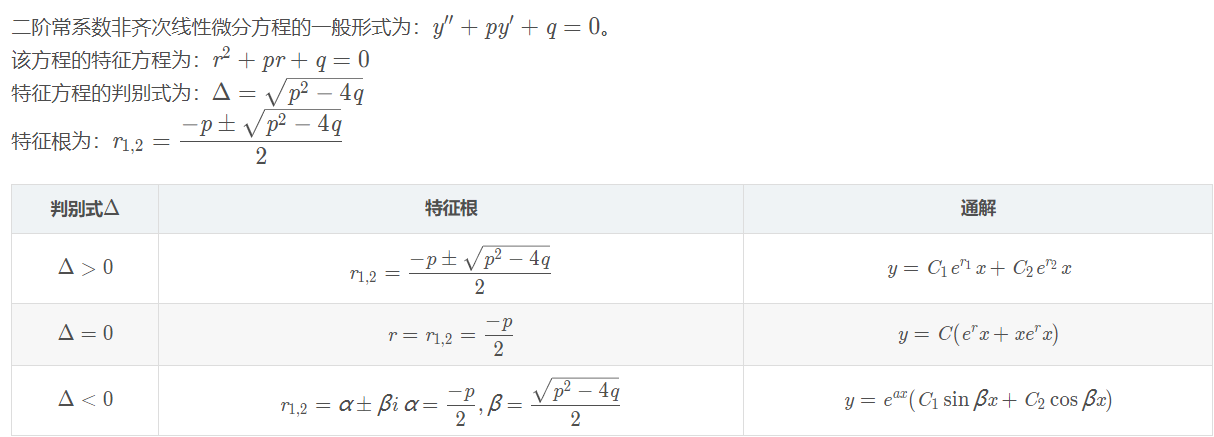2、二阶常系数非齐次线性微分方程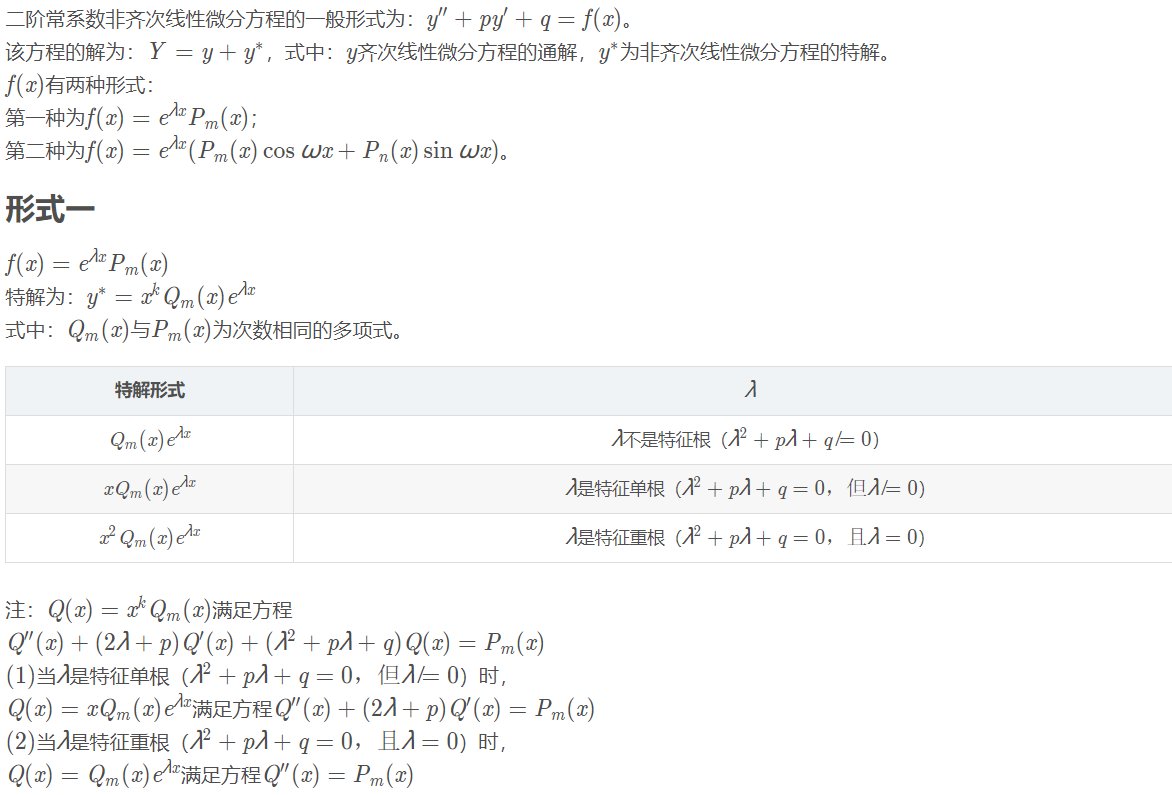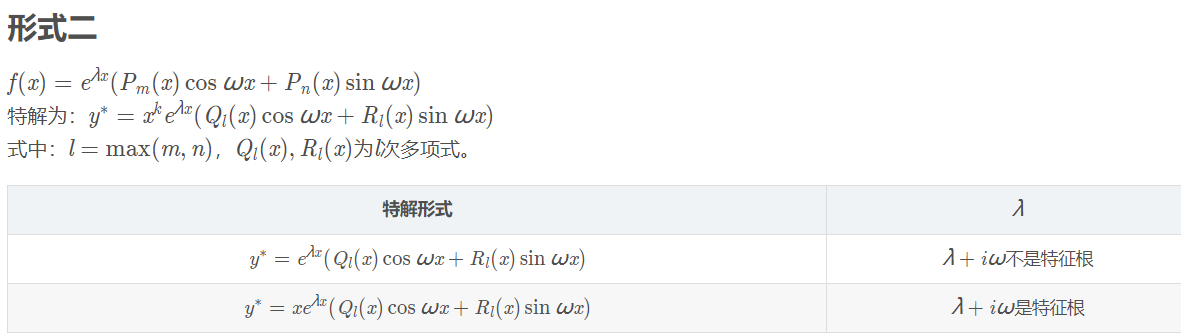展开全文• （建议阅读原文）预备知识　二阶常系数齐次微分方程结论 在二阶常系数齐次微分方程的右端加上一个函数 ， 就得到了二阶常系数非齐次微分方程 这就是二阶常系数非齐次微分方程．其解为 其中 可以写成二阶行列式 其中 ...
（建议阅读原文）预备知识　二阶常系数齐次微分方程结论 　　 在二阶常系数齐次微分方程的右端加上一个函数， 就得到了二阶常系数非齐次微分方程这就是二阶常系数非齐次微分方程．其解为其中可以写成二阶行列式其中都是的函数，后面的括号和自变量被省略．和是对应齐次方程的两个线性无关的解． 应用推导 　　 下面介绍的方法叫常数变易法，其主要思想可参考一阶线性非齐次微分方程的通解  　　 设通解的形式为其中，也是关于的函数． 对该式两边求导，得为了接下来计算方便，我们规定，满足关系1把式 7  代入式 6 ， 得到继续对求导，得到把式 5  式 8  式 9  代回原方程式 1  得化简，得由于和都是式 4  的解，式（9）化为总结一下，刚刚的推导说明，和在（5）的假设条件下，只要满足（10）即可满足（1）式．联立（5）和（10）式，得到关于和的方程组解得其中对（13）的两条式子积分，即可得到（15）（16）代入（5）式，得到方程（1）的解为由于上式满足线性微分方程解的结构，所这已经是通解了．但是必须注意，根据常数变易法，我们只能在没有零点的区间内找到方程式 1  的通解．  1. 这么规定会不会丢失一部分解呢？或许会，但是由于我们已经有了式 1  对应的齐次解和， 根据线性微分方程解的结构（见同济大学的《高等数学》），只需要找到式 1  的任意一个解，就可以找到它的通解．
展开全文• 二阶常系数线性微分方程是形如y''+py'+qy=f(x)的微分方程，其中p，q是实常数。 自由项f(x)为定义在区间I上的连续函数，即y''+py'+qy=0时，称为二阶常系数齐次线性微分方程。若函数y1和y2之比为常数，称y1和y2是线性...


前言：这一部分，我觉得应该是专接本里最难的吧，在学校上高数课的时候就听高数老师说这一部分特别难，对于应付期末考试而言就不讲了，感兴趣的自己研究。到了第一次上专接本课程，老师说第一遍听不打算给你们讲，等你们听两三遍之后我再给你们细讲，事实证明老师说的是对的，到了真正开始讲的时候，一头雾水，这都什么鬼啊？？？什么λ,p,q,k,y*，还分三种情况，计算过程还那么长，学个毛线。在相当长的一段时间内只会了第一种情况，到了大三没办法再不学就晚了，然后才慢慢会的，结果今年考试，貌似就出了一个填空题。我也不知道考研这一部分是不是重点，只想说，会了就不怕它出，为什么花大段文字说它难，是真难，建议找个大的时间空挡期，来慢慢啃这块“硬骨头”。我想花两次或者三次把这一题型讲完，一下子灌输接受不了且效果不好，况且我这书上做了很多笔记，万一哪天这本专接本书丢了岂不是很可惜，废话少说，直接上题。正文：什么是二阶常系数线性微分方程？二阶常系数线性微分方程是形如y''+py'+qy=f(x)的微分方程，其中p，q是实常数。自由项f(x)为定义在区间I上的连续函数，即y''+py'+qy=0时，称为二阶常系数齐次线性微分方程。若函数y1和y2之比为常数，称y1和y2是线性相关的；若函数y1和y2之比不为常数，称y1和y2是线性无关的。特征方程为：r²+pr+q=0，然后根据特征方程根的情况对方程求解。什么是特征方程？特征方程是为研究相应的数学对象而引入的一些等式，它因数学对象不同而不同，包括数列特征方程、矩阵特征方程、微分方程特征方程、积分方程特征方程等等。例如y''+3y'+2y=0的特征方程就是r²+3r+2=0齐次线性微分方程的通解分哪几种情况？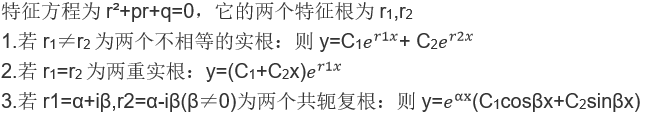可利用求根公式来解特征根，步骤如下1.化方程为一般式：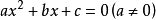2.确定判别式，计算Δ(希腊字母，音译为戴尔塔)。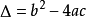；3.若Δ>0，该方程在实数域内有两个不相等的实数根：；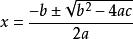若Δ=0，该方程在实数域内有两个相等的实数根: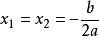；  若Δ<0，该方程在实数域内无解，但在虚数域内有两个共轭复根，为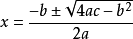。接下来每个情况举个例子供大家参考例题1：(对应情况1)求微分方程y"-3y'+2y=0的通解．解：y"-3y'+2y=0，特征方程为 r2-3r+2=0，(r-1)(r-2)=0．特征根为 r1=1，r2=2．方程的通解为 y=C1ex+C2e2x．例题2：(对应情况2)微分方程y″+6y′+9y=0的通解y=______．解：λ2+6λ+9=0，求解可得，λ1，2=-3，从而方程的两个线性无关的解为：e-3x，xe-3x．由二阶齐次线性微分方程解的结构定理可得，所求方程的通解为：y=C1e-3x+C2xe-3x．故答案为：C1e-3x+C2xe-3x．例题3：(对应情况3)r²+r+1=0,b²-4ac<0r=-1/2±√3i/2,有一对共轭复根,实部α=-1/2,虚部β=±√3/2∴ 微分方程通解为：y=e^(-x/2)[C1cos( √3x/2)+C2sin(√3x/2)].明天的内容才是重头戏，非齐次方程特解的求法(待定系数法)，计算过程会相当的长，希望大家一定不要错过。Last but not least:这么长时间以来，谢谢大家的支持，在公众号人数已经91人啦，超过100搞个小活动比如抽奖之类的蛮好,暂时还没想好什么规则，什么礼品，不妨私信给我出出主意。如果觉得文章还不错，点个关注打赏分享再走呗原创不易，且行且珍惜，笔耕不辍，有你支持


展开全文• 二阶常系数齐次常微分方程，指的就是那些形如y''+py'+qy=0的微分方程如果是形如y''+py'+qy=f(x)的微分方程，就叫做二阶常系数线性微分方程顾名思义，就是有二阶导数的微分方程，且p、q是实数下面给出一道例题，能够...
• 二阶常系数线性微分方程是形如y''+py'+qy=f(x)的微分方程，其中p，q是实常数。自由项f(x)为定义在区间I上的连续函数，即y''+py'+qy=0时，称为二阶常系数齐次线性微分方程。若函数y1和y2之比为常数，称y1和y2是线性...
• *文中的微分方程均指代二阶常系数线性微分方程 二阶常系数齐次线性微分方程的形式为： ay′′+by′+cy=0ay″+by′+cy=0ay'' + by' + cy = 0 由于是二阶线性微分方程，所以它有两个解，记为y1、y2y1、y2y_1、y...高等数学
• 线性常系数齐次方程 对于函数 ，有 合理猜测这将是该齐次方程解的形式，将其代入原微分方程（ ），得到特征方程： 该方程的根 和 称为特征根。特征根的讨论分为三种情况： 特征根为两个相异的实根 那么 都是该微分...
• 下面的微分方程，为二阶常系数齐次线性微分方程微分方程与特征方程当特征方程的解为两个不同的实根时，微分方程的通解为：若为两个相同重根：若为共轭虚根：但这些都是怎么来的呢，为何要用特征方程来辅助研究呢？...
• 原文：https://www.q-math.com/?p=282
• 二阶常系数齐次线性微分方程求解方法 第六节 二阶常系数齐次线性微分方程 教学目的：使学生掌握二阶常系数齐次线性微分方程的解法，了解二阶常系数非齐次线性微分方程的解法 教学重点：二阶常系数齐次线性微分方程的...
• 12.9二阶常系数齐次线性微分方程1. 二阶常系数齐次线性微分方程的标准形式 (为常数)2. 特征方程法称为微分方程的特征方程,其根称为特征根，微分方程的通解有三种情况：(1)设特征根为则方程的通解为(2)特征根则方程...
• 在考研数学中，微分方程是一个重要的章节，每年必考，其中的二阶常系数齐次线性微分方程是一个基本的组成部分，它也是求解二阶常系数非齐次线性微分方程的基础，但很多同学对其求解公式不是十分理解，做题时也感到...
• 二阶常系数非齐次线性微分方程的通解 二阶常系数非齐次线性微分方程的形式为： ay″+by′+cy=f(x) 微分方程的通解 = 对应的二阶常系数齐次线性微分方程通解 + 自身的一个特解 简单记为：通解 = 齐次通解 + ...
• 二阶常系数非齐次微分方程的复数解法二阶常系数非齐次微分方程的复数解法二阶常系数非齐次微分方程的复数解法二阶常系数非齐次微分方程的复数解法
• 成考专升本：二阶常系数线性微分方程的考点！　若函数y₁,y₂为该方程两个线性无关的解，即y₁≠ky₂，则该方程的通解为y=C₁y₁+C₂y₂.　考点2 二阶常系数线性非齐次方程y”+py'+qy=f(z)解的结构　若y*为方程y”+...高数复习资料
• 1、二阶常系数齐次线性微分方程的解法 　y''+py'+qy = 0（其中p，q为常数）的方程称为二阶常系数齐次线性微分方程，求解步骤： 　（1）特征方程：λ2+pλ+q = 0; 　（2）根据特征方程的根分为以下三种情形： ...
• 所以，微分方程的实函数解为， y = e^[x+b]*e^(2ix) + e^[x+b]*e^(-2ix) = e^[x+b][e^(2ix)+e^(-2ix)] = 2e^[x+b][cos(2x)] 或 y = e^[x+b]*e^(2ix) - e^[x+b]*e^(-2ix) = e^[x+b][e^(2ix)-e^(-2ix)] = 2e^[x+b][sin...
• 高数的全部知识点到这里就要结束了也许对你们来说是种解脱但对姑姑来...未来可期大结局就该有大结局的样子，高数最后的垂死挣扎，怎能不给你们留下深刻的记忆，今天要学习的“二阶常系数非线性齐次微分方程”，嗯，...
• 高数的全部知识点到这里就要结束了也许对你们来说是种解脱但对姑姑来...未来可期大结局就该有大结局的样子，高数最后的垂死挣扎，怎能不给你们留下深刻的记忆，今天要学习的“二阶常系数非线性齐次微分方程”，嗯，...
• 二阶常系数齐次线性微分方程 方程：y′′+py′+qy=0y{''}+py{'}+qy=0y′′+py′+qy=0 称为二阶常系数齐次线性微分方程, 其中p qp\ qp q均为常数 如果y1 y2y_1\ y_2y1​ y2​是方程的两个线性...
• 这是二阶微分方程，可以求得通解。 这里的常数是有意义的，表示Z=0处入射电压和反射电压。 信号传输入点我们一般认为在z<0处， Assume that an incident wave of the form V0+e−γzV_0^+e^{-{\gamma}z}V0+​e...搜索引擎
• 二阶常系数线性微分方程 定义 齐次方程解法 拉氏变换基础知识 定义 典型函数的拉氏变换 积分下限 性质 反变换 note: 通解+特解 拉氏变换 状态空间 /矩阵方法 二阶常系数线性微分方程 资源：二阶常...
• 一、二阶线性微分方程的引入 【例1】设有一弹簧，它的上端固定，下端挂一个质量为的物体。当物体处于静止状态时，作用在物体上的重力与弹性力大小相等，方向相反。这个位置就是物体的平衡位置。如图，取轴铅直向下...高等数学...